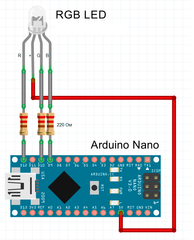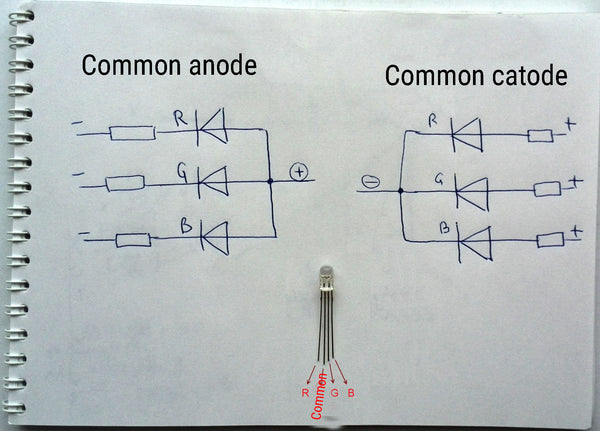# 💡 How to connect RGB LED to Arduino

RGB LED - these are three LEDs of different colors (Red - red, Green - green, Blue - blue), enclosed in one package. Let's see how to connect the RGB LED to the Arduino.

Instructions for connecting the RGB LED to the Arduino
We will need:
• Arduino UNO or another compatible card;
• RGB LED;
• 3 resistors of 220 ohms (here is an excellent set of resistors of the most common denominations);
• connecting wires (I recommend this set);

The difference between RGB LEDs with a common anode and a common cathode

RGB LEDs are of two types: with a common anode (“plus”) and a common cathode (“minus”). The figure shows the schematic diagrams of these two types of LEDs.
The long leg of the LED is always the common power pin.
Separately, the red LED output (R) is located, green (G) and blue (B) are located on the other side of the common output, as shown in the figure. In this article, we will consider connecting the RGB LED with both a common anode and a common cathode.Connect RGB LED with the common anode to Arduino

The connection diagram of the RGB LED with a common anode is shown in the figure. The anode is connected to the "+5 V" on the Arduino board, the other three outputs - to arbitrary digital pins.Please note that we connect each of the LEDs through its own resistor, and do not use one common. It is desirable to do this because each of the LEDs has its own efficiency. And if you connect them all through a single resistor, the LEDs will glow with different brightness.

To quickly calculate the value of the resistor, suitable for your chosen LED, you can use the online calculator for calculating the LEDs.

Control RGB LEDs with Arduino

Rewrite the classic blink sketch. We will enable and disable in turn each of the three colors. Notice that the LED lights up when we apply a low level (LOW) to the corresponding Arduino pin.

```const int pinR = 12;
const int pinG = 10;
const int pinB = 9;

void setup() {
pinMode(pinR, OUTPUT);
pinMode(pinG, OUTPUT);
pinMode(pinB, OUTPUT);
}

void loop() {
digitalWrite(pinR, LOW);
delay(100);
digitalWrite(pinR, HIGH);
delay(200);

digitalWrite(pinG, LOW);
delay(100);
digitalWrite(pinG, HIGH);
delay(200);

digitalWrite(pinB, LOW);
delay(100);
digitalWrite(pinB, HIGH);
delay(200);
}```

Assemble the circuit on the breadboard

Let's look in action at the blinking RGB LED. The LED, in turn, lights up in red, green and blue. Each color burns for 0.1 seconds, and then goes out for 0.2 seconds, and the next one turns on.
You can light each channel separately, you can all at the same time, then the color of the glow will change.Connect RGB LED with a common cathode to Arduino

If you use an RGB LED with a common cathode, then connect the long LED output to the GND Arduino board and the R, G and B channels to the Arduino digital ports. It should be remembered that the LEDs light up when applying to the channels R, G, B high level (HIGH), in contrast to the LED with a common anode.If you do not change the above sketch, then each color of the LED, in this case, will light 0.2 seconds, and the pause between them will be 0.1 seconds.